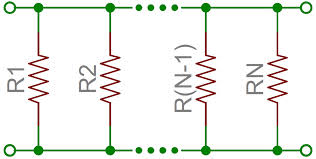Parallel ResistorsAs shown in the figure above, $n$ resistors $R_1,R_2,R_3,\cdots,R_n$ each having equal resistance $R$ are connected in parallel. Find the effective resistance of the whole network.

×